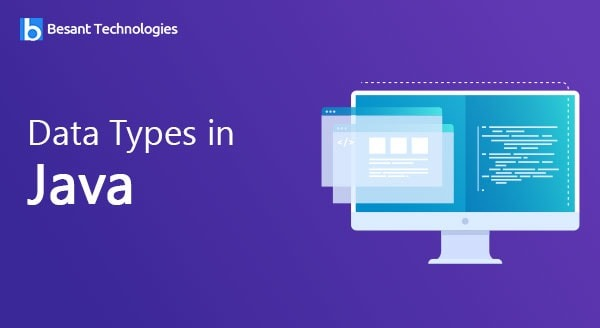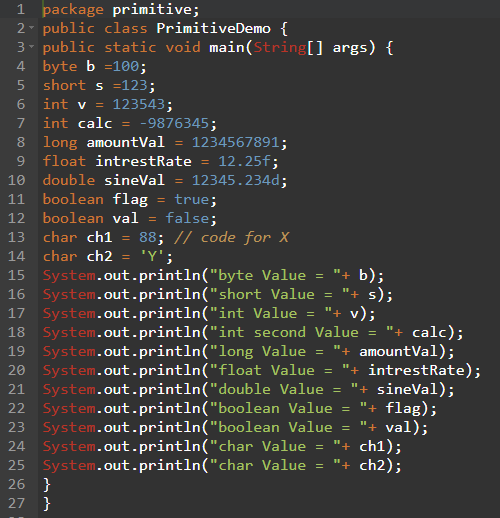Our Special Offer - Get 3 Courses at 24,999/- Only. Read more
Hire Talent (HR):+91-9707 240 250

# General# Data Types in Java

## Data Types in Java

• Data Types can be defined as the set of values which can be stored in a variable along with the operations that can be perform defined ed on those values.
• Data types are used to identify the type data.Primitive Type Size Minimum Value Maximum Value Wrapper Type char 16-bit Unicode 0 Unicode 216-1 Character byte 8-bit -128 +127 Byte short 16-bit -215 (-32,768) +215-1 (32,767) Short Int 32-bit -231 (-2,147,438,648) +231-1 (2,147,483,647) Integer long 64-bit -263 (-9,223,372,036,775,808) +263-1 (9,223,372,036,854,775,807) Long Float 32-bit Approx range 1.4e-045 to 3.4e+038 Float Double 64-bit Approx range 4.9e-324 to 1.8e+308 Double boolean 1-bit true or false Boolean
[/su_table]

Example program to demonstrate the data types.### Integer Types

#### Byte

It can store Whole Number from -128 to 127.

Example:

```byte myNum = 100;
System.out.println(myNum);```

#### Short

It can Store whole number from -32768 to 32767

Example:

```short myNum = 5000;
System.out.println(myNum);

```

#### Int

It can Store whole number -214748648 to 214783647. In general, and in our tutorial, In Our data type is preferred data type when we creat variable with a numeric Value.

Example:

```int myNum = 100000;
System.out.println(myNum);```
##### Long

It can Store whole numbers -922337203685775808 to 9223372036854775807. This is used when int is not large enough to store the value.

Note: That we should end the value with an ‘L’.

Example:

```long myNum = 15000000000L;
System.out.println(myNum);

```

### Floting Point Types

we should use a floating-type whenever we need a number with a decimal, such as 9.99 or 3.14515

#### Flot

It can store fractional numbers from 3.4e-038 to 3.4e+038.

Note: That we should end the value with an ‘F’

Example:

```float myNum = 5.75f;
System.out.println(myNum);

```

### Double

It can store fractional numbers from 1.7e-308to1.7e+038

Note: That we should end the value with a ‘d’:

Example:

```double myNum = 19.99d;
System.out.println(myNum);```

### Scientific Numbers

Floating-Point number can be scientific number with ‘e’ to indicate the power of 10:

Example:

```float f1 = 35e3f;
double d1 = 12E4d;
System.out.println(f1);
System.out.println(d1);```

### Booleans

A boolean data type is declared with the Boolean Keywords and can only allow the values of false:

Example:

```boolean isJavaFun = true;
boolean isFishTasty = false;
System.out.println(isJavaFun);     // Outputs true
System.out.println(isFishTasty);   // Outputs false

```

Boolean Values are mostly used for conditional testing.

### Characters

It is used to store a single character. The character must be bounded by single quotes, like ‘A’ or ‘c’:

Example:

```char myGrade = 'B';

```

Alternatively, we can use ASCII value to display certain Characters:

Example:

```char a = 15, b = 61 c = 65;
System.out.println(a);
System.out.println(b);
System.out.println(c);```

### Strings

It is used to store a sequence of characters(text). String values must be surrounded by double quotes:

Example:

```String greeting = "Hello Besant Technologies";
System.out.println(greeting);

```

A string in Java is really a non-premitive data type, because it refer to an object